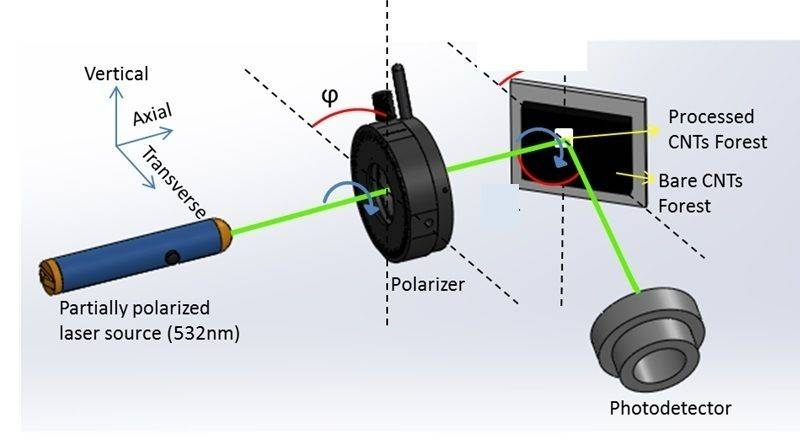# Question on Fresnel Equation

• A
tanveers
Hi All,

I am new to this forum. We are researching light reflection from carbon nanotubes. Now I know about the Fresnel Equation that can be used to determine reflectance for S (perpendicular to the plane of the incident) and P (parallel to the plane of the incident) polarized light.

Now, if I want to know reflectance from a dielectric interface of light beam which is neither S nor P polarized rather it is in between. I would like to make it clearer. Please refer to the attached figure which shows our experimental setup. Now if we change the angle φ between 0 and 90 degree then they are basically representing P and S polarization respectively and the reflectance from the sample can be predicted using Fresnel equation. However if the variation of φ is continuous from 0 to 90 degree how do I predict the reflectance? Lets say φ = 10, 20, 30,…..90. Thanks a lot in advance.